cancel
Showing results for
Did you mean:Frequent Visitor

## Year to date average in the total of a headcount table

Hello, I have a table with the monthly terminations Jan to Sep. 2022 and the respective monthly headcount (HC). I need both KPI's to calculate the turn-over rate. The monthly turn-over rate works but in the total the value is wrong. The sum of headcount doesn't make sense, I need the average instead.

That's why I'm searching for the possibility to get in the total of the HC column the average headcount instead of the sum. Some ideas?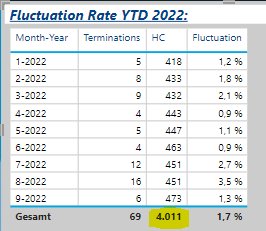1 ACCEPTED SOLUTIONCommunity Support

Hi @Werfuks ,

``HC = DIVIDE(CALCULATE(SUM('Table'[Headcount]),ALLEXCEPT('Table','Table'[Month/Year])),DISTINCTCOUNT('Table'[Month/Year]))``

Output:Best Regards,

Jianbo Li

If this post helps, then please consider Accept it as the solution to help the other members find it more quickly.

9 REPLIES 9Community Support

Hi @Werfuks ,

``HC = DIVIDE(CALCULATE(SUM('Table'[Headcount]),ALLEXCEPT('Table','Table'[Month/Year])),DISTINCTCOUNT('Table'[Month/Year]))``

Output:Best Regards,

Jianbo Li

If this post helps, then please consider Accept it as the solution to help the other members find it more quickly.Solution Sage

Hi @Werfuks ,

try thisIf this post helps, then please consider Accept it as the solution to help the other members find it more quickly.Community Support

Hi @Werfuks ,

Based on your description, I have created a simple sample: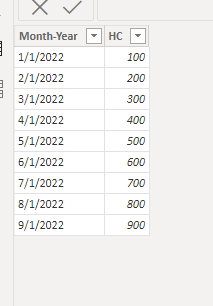``Measure = IF(ISINSCOPE('Table'[HC]),SUM('Table'[HC]),AVERAGE('Table'[HC]))``

Final output: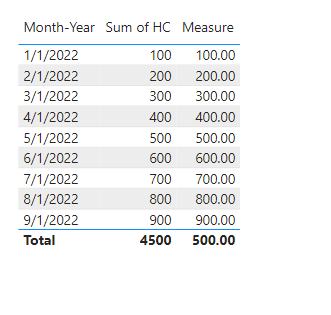Best Regards,

Jianbo Li

If this post helps, then please consider Accept it as the solution to help the other members find it more quickly.Frequent Visitor

Hello, thank you very much for your proposal. I guess I should have explained the basis of my data a bit more detailed. Sorry for that!

I don't have a table like this:My basis table is like this for round about 17000 employees for every month: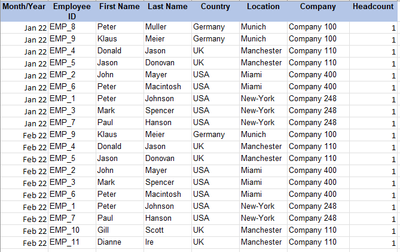The dashboard use two slicers. One for the country and one for location which should help the user to see how much headcount in total he has in a selected country or in a selected location eg. in Feb 2022 and what the YTD average is.

With my basis table I was not able to calculate an average. Average was always 1.

As a workaround I tried to create a new agregated table based on this basis table by using the summarize function with regards to the country. The total and the average worked with regards to the country, if the user select only a country but not if the user select a location because my summarize function is only summarizing countries. At this stage I am not sure if I can create a table based on the slicers or if I think much to difficult and don't see the easy solution? Best regards ChristianSolution Sage

I share with you my pbi sample file:

https://1drv.ms/u/s!Aj45jbu0mDVJiHRJvAMfvPPe444G?e=LiXc8R

If this post helps, then please consider Accept it as the solution to help the other members find it more quickly.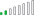Resolver II

Hello @Werfuks

you can take another table and do not consider or add month there and you can consder average in the valueFrequent Visitor

Hi mangaus111, if I use this possibility I receive always "1". In the months and in the total.Solution Sage

Otherwise you can simply select from here the average, instead of the sum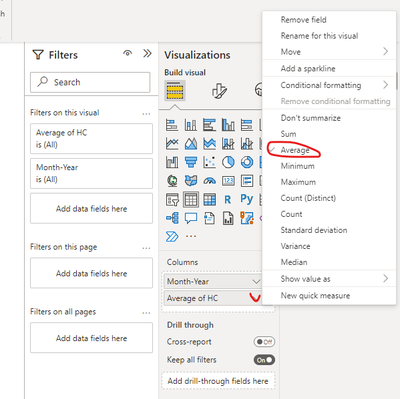If this post helps, then please consider Accept it as the solution to help the other members find it more quickly.Solution Sage

Hi @Werfuks ,

use thie measure:

AVERAGE = AVERAGE('Table'[HC])

If this post helps, then please consider Accept it as the solution to help the other members find it more quickly.